Please wait a minute...
 Chin. Phys. B, 2020, Vol. 29(11): 114701    DOI: 10.1088/1674-1056/aba612
 ELECTROMAGNETISM, OPTICS, ACOUSTICS, HEAT TRANSFER, CLASSICAL MECHANICS, AND FLUID DYNAMICS Prev   Next

# Dynamical interactions between higher-order rogue waves and various forms of n-soliton solutions (n → ∞) of the (2+1)-dimensional ANNV equation

Md Fazlul Hoque1, †, Harun-Or-Roshid1,, ‡, and Fahad Sameer Alshammari2
1 Department of Mathematics, Pabna University of Science and Technology, Pabna 6600, Bangladesh
2 Department of Mathematics, College of Science at Alkharj, Prince Sattam bin Abdulaziz University, Alkharj, Saudi Arabia
 Abstract  We present new lemmas, theorem and corollaries to construct interactions among higher-order rogue waves, n-periodic waves and n-solitons solutions (n → ∞) to the (2+1)-dimensional asymmetric Nizhnik–Novikov–Veselov (ANNV) equation. Several examples for theories are given by choosing definite interactions of the wave solutions for the model. In particular, we exhibit dynamical interactions between a rogue and a cross bright-dark bell wave, a rogue and a cross-bright bell wave, a rogue and a one-, two-, three-, four-periodic wave. In addition, we also present multi-types interactions between a rogue and a periodic cross-bright bell wave, a rogue and a periodic cross-bright-bark bell wave. Finally, we physically explain such interaction solutions of the model in the 3D and density plots. Keywords:  the (2+1)-dimensional asymmetric Nizhnik-Novikov-Veselov (ANNV) equation      higher-order rogue waves      n-solitons      periodic waves      bright-dark bell waves Received:  01 June 2020      Revised:  06 July 2020      Accepted manuscript online:  15 July 2020 Corresponding Authors:  †Corresponding author. E-mail: fazlul_math@yahoo.co.in; f.alshammari@psau.edu.sa ‡Corresponding author. E-mail: harunorroshidmd@gmail.com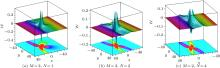Fig. 1.  The 3D (upper) and density (lower) profiles of Eq. (8) for ${\mathscr{W}}$: interaction between (a) a rogue and a bright bell wave, (b) a rogue and a two-bell (one bright and one dark) wave, and (c) a rogue and a four-bell (double bright and double dark) wave.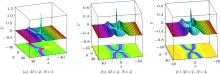Fig. 2.  The 3D (upper) and density (lower) profiles of Eq. (8) for ${\mathscr{V}}$: interaction between (a) a rogue and a bright bell wave, (b) a rogue and a two-cross-bright-bell wave, and (c) a rogue and a four-cross-bright-bell wave.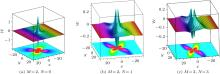Fig. 3.  The 3D (upper) and density (lower) profiles of Eq. (17) for ${\mathscr{W}}$: (a) a single rogue wave; and interaction between (b) a rogue and a bright bell wave, and (c) a rogue and a three-bell (double bright and single dark) wave.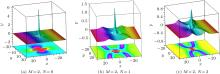Fig. 4.  The 3D (upper) and density (lower) profiles of Eq. (17) for ${\mathscr{V}}$: (a) a single rogue wave; and interaction between (b) a rogue and a cross-bright bell wave, and (c) a rogue and a triple cross-bright bell wave.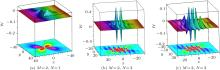Fig. 5.  The 3D (upper) and density (lower) profiles of Eq. (18) for ${\mathscr{W}}$: interaction between (a) a rogue and a periodic wave, (b) a rogue and a double periodic wave, and (c) a rogue and a triple periodic wave.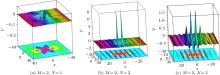Fig. 6.  The 3D (upper) and density (lower) profiles of Eq. (18) for ${\mathscr{V}}$: interaction between (a) a rogue and a periodic wave, (b) a rogue and a double periodic wave, and (c) a rogue and a triple periodic wave.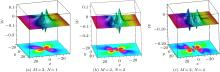Fig. 7.  The 3D (upper) and density (lower) profiles of Eq. (19) for ${\mathscr{W}}$: interaction between (a) a rogue and a periodic wave, (b) a rogue and a double periodic wave, and (c) a rogue and a four-periodic-bell wave.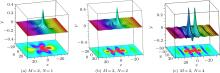Fig. 8.  The 3D (upper) and density (lower) profiles of Eq. (19) for ${\mathscr{V}}$: interaction between (a) a rogue and a periodic wave, (b) a rogue and a double-periodic-bell wave, and (c) a rogue and a four-periodic-bell wave.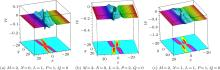Fig. 9.  The 3D (upper) and density (lower) profiles of Eq. (20) for ${\mathscr{W}}$: multi-interaction among a rogue periodic wave and bright-bark bell soliton waves.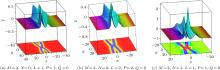Fig. 10.  The 3D (upper) and density (lower) profiles of Eq. (20) for ${\mathscr{V}}$: multi-interaction among a rogue periodic wave and bright-bark bell soliton waves.